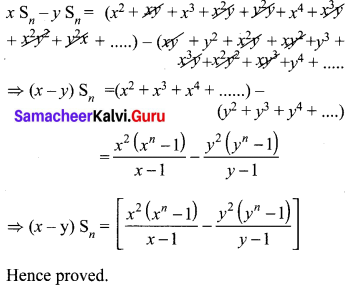# Samacheer Kalvi 10th Maths Solutions Chapter 2 Numbers and Sequences Ex 2.8

## Tamilnadu Samacheer Kalvi 10th Maths Solutions Chapter 2 Numbers and Sequences Ex 2.8

10th Maths Exercise 2.8 Question 1.
Find the sum of first n terms of the G.P.
(i) 5, -3, $$\frac { 9 }{ 5 }$$, – $$\frac { 27 }{ 25 }$$, ……….
(ii) 256, 64, 16, ……..
Solution:
(i) 5, -3, $$\frac { 9 }{ 5 }$$, $$\frac { -27 }{ 25 }$$, ………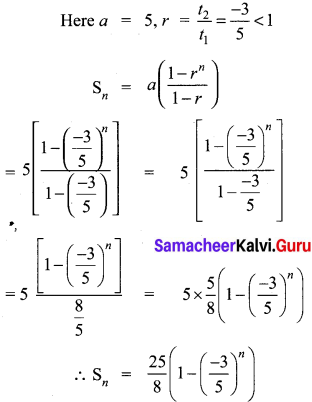(ii) 256, 64, 16,…….
a = 256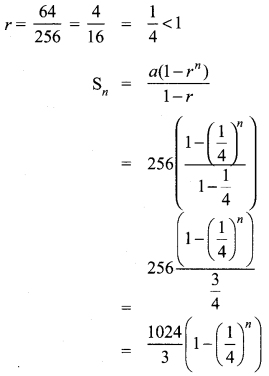Exercise 2.8 Class 10 Maths Solution Question 2.
Find the sum of first six terms of the G.P. 5, 15, 45, …
Solution:
G.P.= 5, 15, 45, 15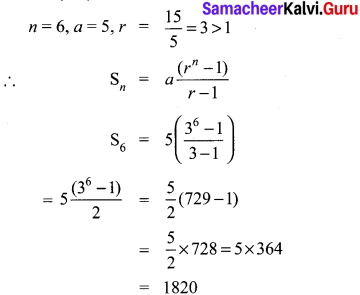Ex 2.8 Class 10 Samacheer Question 3.
Find the first term of the GP. whose common ratio 5 and whose sum to first 6 terms is 46872.
Solution:
Common ratio, r = 5
S6 = 46872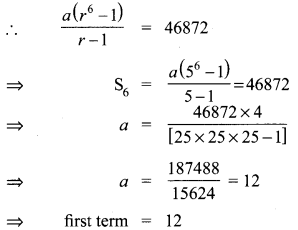10th Maths Exercise 2.8 Samacheer Kalvi Question 4.
Find the sum to infinity of (i) 9 + 3 + 1 + ….
(ii) 21 + 14 + $$\frac { 28 }{ 3 }$$ + ….
Solution:
(i) 9 + 3 + 1 + ….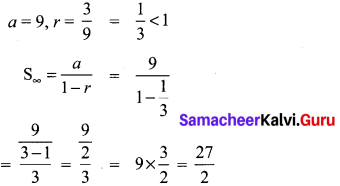(ii) 21 + 14 + $$\frac { 28 }{ 3 }$$ + ….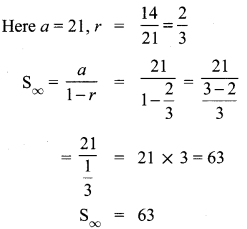10th Maths Exercise 2.8 In Tamil Question 5.
If the first term of an infinite G.P. is 8 and its sum to infinity is $$\frac { 32 }{ 3 }$$ then find the common ratio.
Solution:
a = 8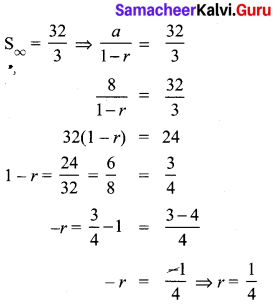Class 10 Maths Exercise 2.8 Solutions Question 6.
Find the sum to n terms of the series
(i) 0.4 + 0.44 + 0.444 +…. to n terms
(ii) 3 + 33 + 333 + …. to n terms
Solution:
(i) 0.4 + 0.44 + 0.444 + … to n terms
= 4 (0.1 + 0.11 + 0.111 + … to n terms)
= $$\frac { 4 }{ 9 }$$ (0.9 + 0.99 + 0.999 + … to n terms)
= $$\frac { 4 }{ 9 }$$ (1 -0.1) + (1 – 0.01) +(1 – 0.001) + … n terms)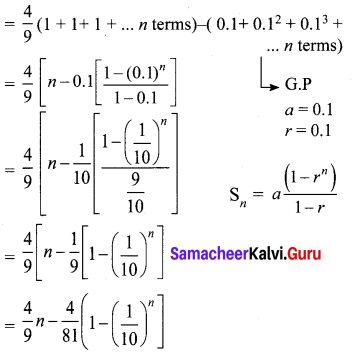(ii) 3 + 33 + 333 + … to n terms.
= 3 (1 + 11 + 111 + …….. to n terms)
= $$\frac { 3 }{ 9 }$$ (9 + 99 + 999 +… to n terms)
$$\frac { 1 }{ 3 }$$ = [(10 – 1) + (100 – 1) + (1000 – 1) + … to n terms]
= $$\frac { 1 }{ 3 }$$ [(10 + 100 + 1000 + .. ) + (-1)n]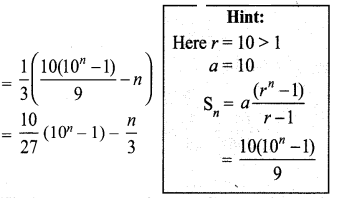2.8 Exercise Class 10 Question 7.
Find the sum of the Geometric series
3 + 6 + 12 + …… + 1536.
Solution:
3 + 6 + 12 + …… + 1536
Here a = 3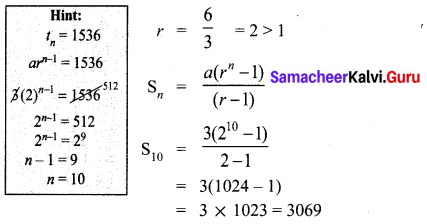Exercise 2.8 Class 10 Maths Question 8.
Kumar writes a letter to four of his friends. He asks each one of them to copy the letter and mail to four different persons with the instruction that they continue the process similarly. Assuming that the process is unaltered and it costs ₹2 to mail one letter, find the amount spent on postage when 8th set of letters is mailed.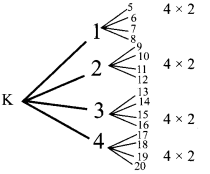Solution:
Kumar (1) 2 × 4
Cost of posting 1st set of Letters.
Cost of posting 2nd set of letters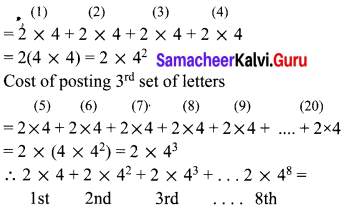Amount spent
[a + ar + ar2 +… arn-1]
= 4 [2 + 2 × 4 + 2 × 42 ÷ + 2 × 47]
= 4 [Sn] Here n = 8, r = 4
It is a G.P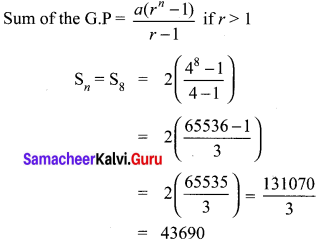∴ Cost of postage after posting 8th set of letters
= 4 × 43690 = ₹ 174760

Ex 2.8 Class 10 Maths Question 9.
Find the rational form of the number 0.$$\overline{123}$$
Solution:
Let x = 0.123123123 ………. ⇒ x = 0.$$\overline{123}$$ …..(1)
Multiplying 1000 on both rides
1000 x = 123.123123… ⇒ 1000x = 123.$$\overline{123}$$ …..(2)
(2) – (1) = 1000x – x 123.$$\overline{123}$$ – 0.$$\overline{123}$$.
⇒ 999 x = 123
⇒ x = $$\frac { 123 }{ 999 }$$
⇒ x = $$\frac { 41 }{ 333 }$$ Rational number

Maths Exercise 2.8 Class 10 Question 10.
If Sn = (x + y)+(x2 + xy + y2) + (x3 + x2y + xy2 + y3) + …n terms then prove that (x – y)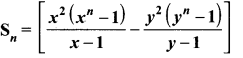Solution:
Sn = (x + y) + (x2 + xy + y2) + (x3 + x2y + xy2 + y3) + …n terms
⇒ x. Sn = (x + y)x + (x2 + xy + y2)x + (x3 + x2y + xy2 + y3) x + ….
⇒ x. Sn = x2 +xy + x3 + x2y + y2x + x4 + x3y
+ x2 y2 + y3 x +…… ….(1)
Multiplying ‘y’ on both sides,
= y.Sn = (x + y)y + (x2 + xy + y2) y+
(x3 + x2y + xy2 + y3) y + ….
= y.Sn = xy + y2 + x2y + xy2 + y3 + x3y
+ x3y + x2y2 + xy3 + y4 + ……….
(1) – (2) ⇒